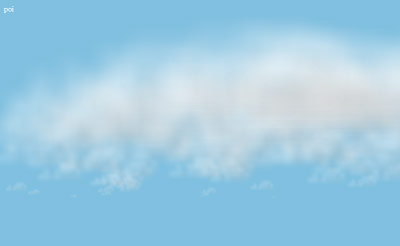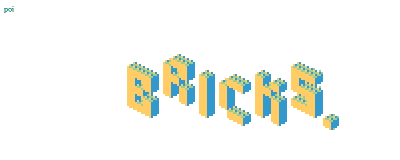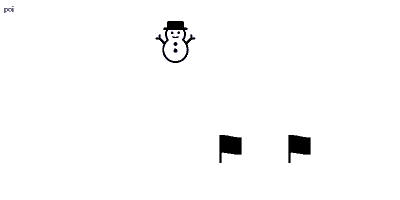# 256B.HTM ROUND 2

539 words ~ 3-5 minsMathieu 'p01' Henri on September 2nd, 2002

# 256b.htm round 2

The second edition of the 256B.HTM contest allowed 3 entries per author. Mine ranked 1st, 2nd and 4th out of 43. Only a few points shy of a total take over!

## 256B.HTM round 2

The first 256B.HTM contest was such a success with 62 entries in February 2002 that it made way for a second edition 6 months later, in August 2002. This time authors were allowed a maximum of 3 entries. My entries ranked 1st, 2nd and 4th out of 43. So close, yet so far.

### Rules

The task was to create a tiny website in 256 bytes, using HTML, JavaScript, CSS, GIF, Flash, SVG, VRML and so on. The main rules where:

1. Total size of all files must be less or equal to 256 bytes.
2. The entry have to run offline in one of the commonly used web browsers ( Internet Explorer 6, Opera 6, Netscape 6 and Mozilla 0.9 ).

1. The code don't have to be valid, cross-browser or DOM compatible.
2. All plugin based technologies are allowed.
3. It's not allowed to use the file name as source code.

## Three different styles

• Clouds a procedural image
• Bricks an interactive building tool
• Ski a frentic game

## 1. Clouds ranked #1

This procedural graphics in less than 256 bytes showing a random cloud scape ranked 1st.### Source code

Here is the current version, working in recent browsers

``<body id=B bgcolor=#7bd text=#fff onload="for(i=99;i--;)B.innerHTML+='<img src=c style=position:absolute;left:'+Math.random()*800+'px;top:'+i*4+'px;width:'+(50-i/2)+'%;opacity:.15>'">poi``

And here is the version submitted back then, that only worked in IE5.5

``<body id=B bgcolor=80c0e text=white onload="for(i=99;i--;)B.innerHTML+='<img src=c style=position:absolute;left:'+Math.random()*800+';top:'+i*4+';zoom:'+(50-i/2)+';filter:alpha(opacity=15)>'">poi``

These weigh 248 and 255 bytes respectively including a 60 bytes big picture.

### How do draw fluffy clouds

The image above is drawn 99 times at low opacity, at a random horizontal position and decreasing size. The accumulation and opacity combined with the size and the blue background of the page make the illusion of fluffy clouds.

## 2. Bricks ranked #2

This tiny LEGO(tm) construction kit ranked #2.Click on the background to summon a brick in the top left corner. Drag'n drop the brick(s) to make any LEGO(tm)ish construction you want i.e. a dinosaur, a castle, a bus, a rocket...

### Source code

Here is the current version, working in recent browsers

``<html onclick='b.appendChild(i=new Image());i.src=4'onmousemove='if(top.i)with(i.style)width=32,position="absolute",left=event.x-8&-8,top=event.y-8&-8'id=b>poi<p>``

And here is the version submitted back then, that only worked in IE5.0

``<body id=B onload=i=0 onclick="B.innerHTML+='<img src=4 width=32 style=position:absolute ondrag=with(this.style)zIndex=++i,left=event.x-8&-8,top=event.y-8&-8>'">poi <br>``

These versions weigh 228 and 238 bytes respectively including a 69 bytes image.

## 3. Ski ranked #4

This frentic skiing game ranked #4Move the little skier with your mouse to pass through as many gates as possibe.

### Source code

Here is the current version, working in recent browsers

``<html id=B onmousemove=x=event.screenX><script>setInterval("P='<p style=position:absolute;font-size:80px;left:';w=--a?w:Math.random(S=x<w||x-w>140?0|alert(S):S+1)*640;B.innerHTML='poi'+P+x+'px>⛄'+P+w+'px;top:'+(a&=63)*2+'%>⚑ ⚑'",x=w=a=S=0)</script>``

And here is the version submitted back then, that only worked in IE5.5

``<body id=B onmousemove=x=event.x><script>P='<p style=position:absolute;font:99webdings;left:';x=w=a=S=0;setInterval("a-=2;if(!(a&=127)){if(x-w<16||x-w>160)S=~alert(S);w=Math.random(++S)*640};B.innerHTML='poi'+P+x+'>‡'+P+w+';top:'+a+'%>w&#32 w'",9)</script>``

These weigh 256 bytes each.

## Closing words

The 256B.HTM contests rocked! They were far ahead of their time to show that 256 bytes can make for a great show on the web. Kudos to the organizers and all the participants!